Question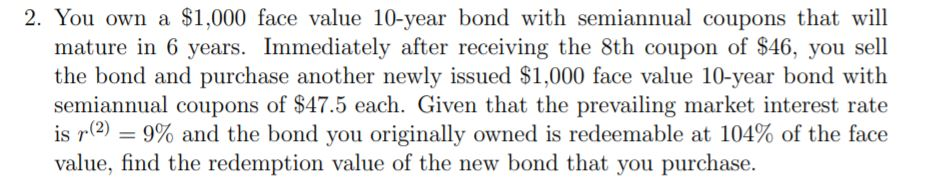Selling price of the original bond is \$ \$ 1,032.71 calculated using PV function of Excel as follows: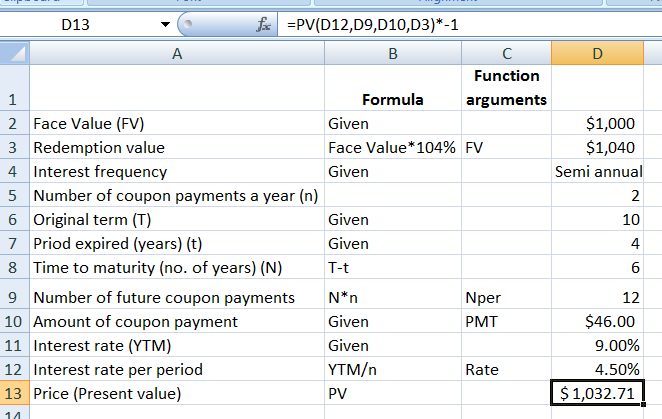Treating this amount as the investment (price), Redemption value of new bond is \$1,000.46 calculated using the FV function of Excel as follows:

#### Earn Coins

Coins can be redeemed for fabulous gifts.

Similar Homework Help Questions
• ### 7. Problem 7: 1. A \$1,000 par value ten-year 8% bond has semiannual coupons. The redemption...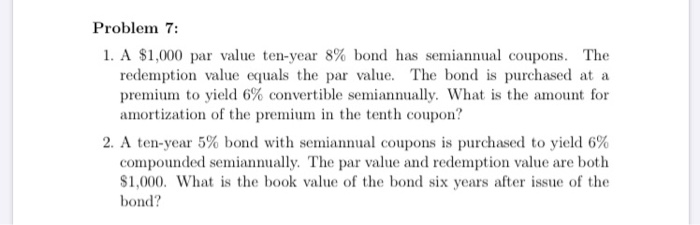7. Problem 7: 1. A \$1,000 par value ten-year 8% bond has semiannual coupons. The redemption value equals the par value. The bond is purchased at a premium to yield 6% convertible semiannually. What is the amount for amortization of the premium in the tenth coupon? 2. A ten-year 5% bond with semiannual coupons is purchased to yield 6% compounded semiannually. The par value and redemption value are both \$1,000. What is the book value of the bond six years...

• ### If a bond with a face value of \$1,000, 8 years to maturity, semiannual coupons, and...

If a bond with a face value of \$1,000, 8 years to maturity, semiannual coupons, and the yield to maturity of 4% sells for \$1,067.89, what is its annual coupon rate? A. 4% B. 5% C. 2% D. 3% E. 2.5%

• ### (2) A \$1,000 seven-year 6% bond with semiannual coupons is redeemable for \$1,065. It was originally...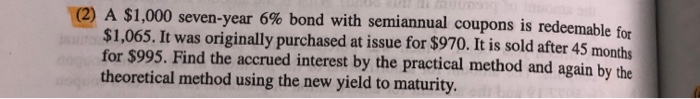(2) A \$1,000 seven-year 6% bond with semiannual coupons is redeemable for \$1,065. It was originally purchased at issue for \$970. It is sold after 45 months for \$995. Find the accrued interest by the practical method and again by the theoretical method using the new yield to maturity.

• ### An 8 percent per year coupon bond with semiannual coupons and \$10,000 face value promises to...

An 8 percent per year coupon bond with semiannual coupons and \$10,000 face value promises to pay: A. \$800 per year and \$10,000 on maturity B. \$80 per year and \$1,000 on maturity C.  \$40 every six months and \$1,000 on maturity D. \$400 every six months and \$10,000 on maturity 2. The Wall Street Journal shows the bid and ask quotes for a T-bill maturing in 60 days as 0.098 and 0.088, respectively. If you want to sell a 60-day...

• ### A coupon bond with a par value of \$1,000 and a 10-year maturity pays semiannual coupons...

A coupon bond with a par value of \$1,000 and a 10-year maturity pays semiannual coupons of \$21. (a) Suppose the yield for this bond is 4% per year compounded semiannually. What is the price of the bond? (b) Is the bond selling above or below par value? Why?

• ### How do you find the PMT? Problem 2 (Bond Valuation) You consider purchasing a \$1,000 face value 5-year semiannual coupon...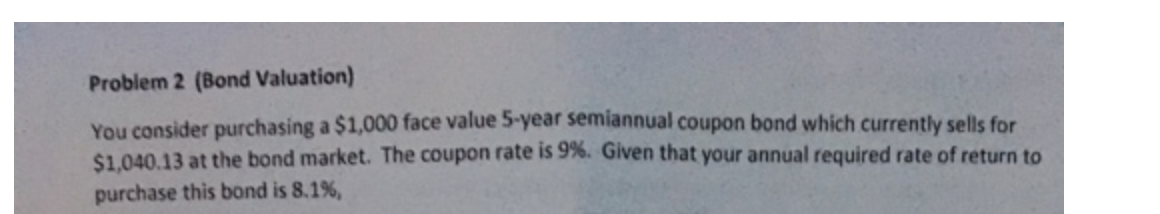How do you find the PMT? Problem 2 (Bond Valuation) You consider purchasing a \$1,000 face value 5-year semiannual coupon hand \$1.000 face value 5-year semiannual coupon bond which currently sells for \$1,040.13 at the bond market. The coupon rate is 9%. Given that your annual required rate of return to purchase this bond is 8.1%,

• ### A \$1,000 seven-year 4% bond with semiannual coupons is redeemable for \$1,052. It was originally purchased at issue for...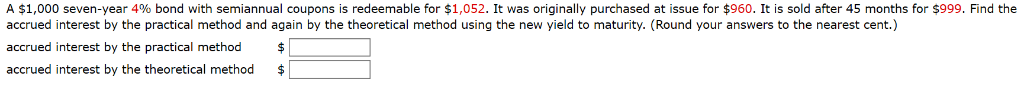A \$1,000 seven-year 4% bond with semiannual coupons is redeemable for \$1,052. It was originally purchased at issue for \$960. It is sold after 45 months for \$999. Find the accrued interest by the practical method and again by the theoretical method using the new yield to maturity. (Round your answers to the nearest cent.) accrued interest by the practical method \$ accrued interest by the theoretical method A \$1,000 seven-year 4% bond with semiannual coupons is redeemable for \$1,052....

• ### A \$1,000 par value 10-year bond with annual coupons is redeemable at \$1,055, and has a...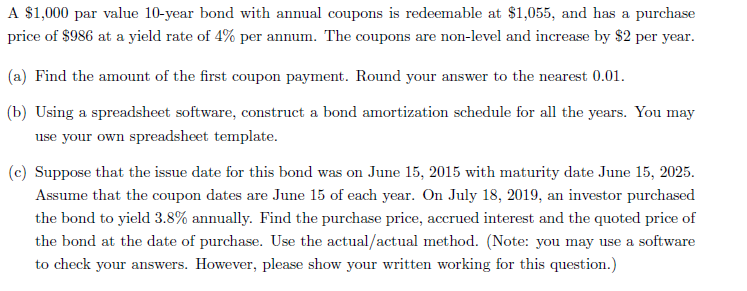A \$1,000 par value 10-year bond with annual coupons is redeemable at \$1,055, and has a purchase price of \$986 at a yield rate of 4% per annum. The coupons are non-level and increase by \$2 per year. (a) Find the amount of the first coupon payment. Round your answer to the nearest 0.01. (b) Using a spreadsheet software, construct a bond amortization schedule for all the years. You may use your own spreadsheet template. (c) Suppose that the issue...

• ### The Federal Government 2-year coupon bond has a face value of \$1,000 and pays annual coupons...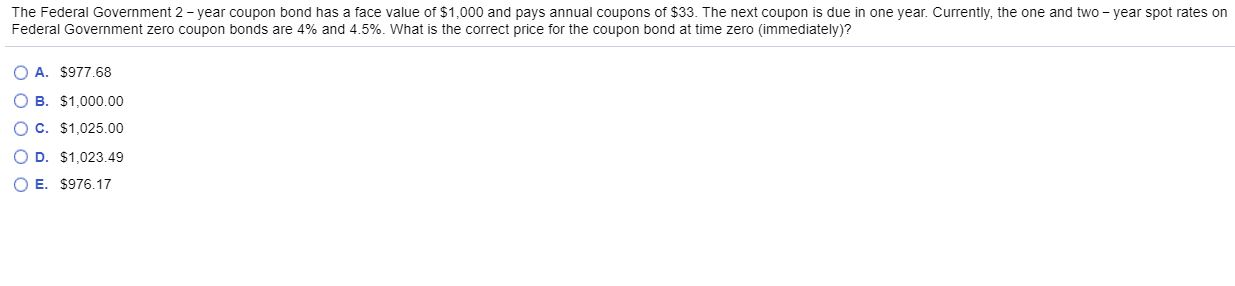The Federal Government 2-year coupon bond has a face value of \$1,000 and pays annual coupons of \$33. The next coupon is due in one year. Currently, the one and two-year spot rates on Federal Government zero coupon bonds are 4% and 4.5%. What is the correct price for the coupon bond at time zero immediately)? O A. \$977.68 O B. \$1,000.00 OC. \$1,025.00 OD. \$1,023.49 E. \$976.17

• ### 2. A 3 year bond has a face value of 1 million, semiannual coupons, a nominal...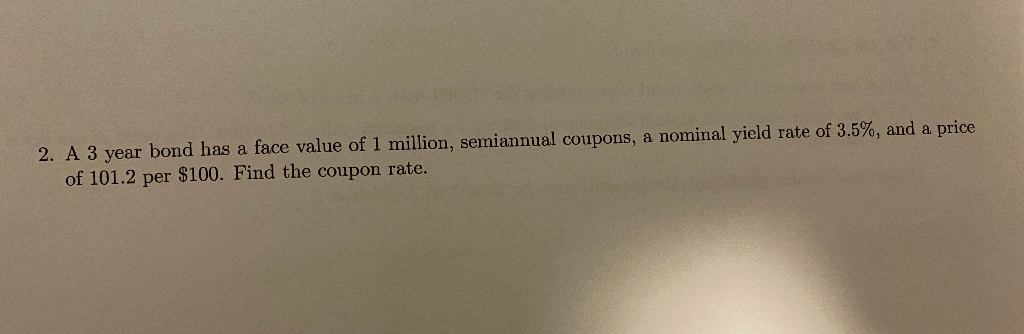2. A 3 year bond has a face value of 1 million, semiannual coupons, a nominal yield rate of 3.5%, and a price of 101.2 per \$100. Find the coupon rate.